MS 095 PreAlgebra: Fractions, decimals, and conversion factors Name:

In this laboratory we will again work with fractions and decimals. Our main goal will be to arrive at two conversion factors. One to convert inches to centimeters, the other to convert centimeters to inches. As a concluding activity we will look at how to convert centimeters to meters.

Activity One: Obtaining conversion factors.

 Reading the inches side of the ruler: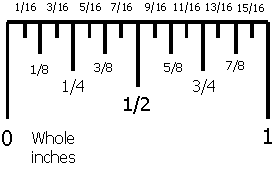Reading the centimeters side of the ruler: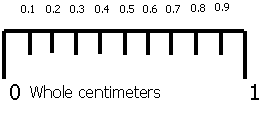Measure the length (or width or height) of five objects using both the inches side of the ruler and the centimeters side of the ruler.

Object

Length in inches to nearest 1/16th of an inch

Length in centimeters to nearest 0.1 centimeter

Inches ÷ Centimeters

Centimeters ÷ Inches

1

2

3

4

5

Averages: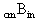: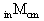:

To convert from inches to centimeters, multiply the inches by.
To convert from centimeters to inches, multiply the centimeters by.

Use your conversion factors to determine the answers to the following questions:

1. _______ If an object is 67½ inches tall, how many centimeters tall is the object?
2. _______ If a bird has a wingspan of 31.2 cm, what is the wingspan in inches?
Official values

The official value foris 2.54 centimeters per inch. In fact, an inch is now officially defined to be exactly 2.54 centimeters. Calculate how close you came to the correct value forby making the following calculation:

Use the formula: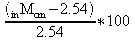, substituting your value forinto the formula. Put a percent (%) sign after your answer: by multiplying by a hundred you have calculated the percentage difference of yourfrom the actual value of 2.54.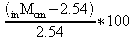= _____________________%

Toughie: ifis 2.54 centimeters per inch, what is the official value for?= _____________

Abbreviations: "in" is the abbreviation for inches, "cm" is the abbreviation for centimeters.

Converting Centimeters to Meters

The metric system is designed to be simply. Since there are one hundred centimeters in a meter, you can convert centimeters to meters by dividing the centimeters by 100. This is the same as moving the decimal point two places to the left.

1. Convert my height, 170.18 centimeters, to meters.
2. Convert the distance around lap four of the PICS track, 43722 cm, to meters. Essentially only the inside edge of lane one is 400 meters. Even the middle of lane one is longer. Lane one is typically around 410 meters, at PICS it is about 413 meters but completely unusable at the moment.

Homework
1. _______ If an object is 107¾ inches tall, how many centimeters tall is the object?
2. _______ If a box is 34⅜ inches wide, how many centimeters wide is the box?
3. _______ If a waterfall is 996 inches high, how many centimeters high is the waterfall?
4. _______ If a pendulum has a length of 50.5 cm, what is the length in inches?
5. _______ If a woman has a waist measurement of 91.4 centimeters, what is the waist measurement in inches?
6. _______ Convert the answer to question three from centimeters to meters.